# COSC 1 P 03 Recursion Recursion Recursion Just

• Slides: 24COSC 1 P 03 Recursion Recursion Recursion Just be thankful you're not getting all the government you're paying for. Will Rogers (1879 -1935) Data Structures and Abstraction 8. 1COSC 1 P 03 Recursion · recursion · in Mathematics - factorial - Fibonacci numbers - define infinite set with finite definition · in Computer Science - syntax rules ° finite definition, infinite language Data Structures and Abstraction 8. 2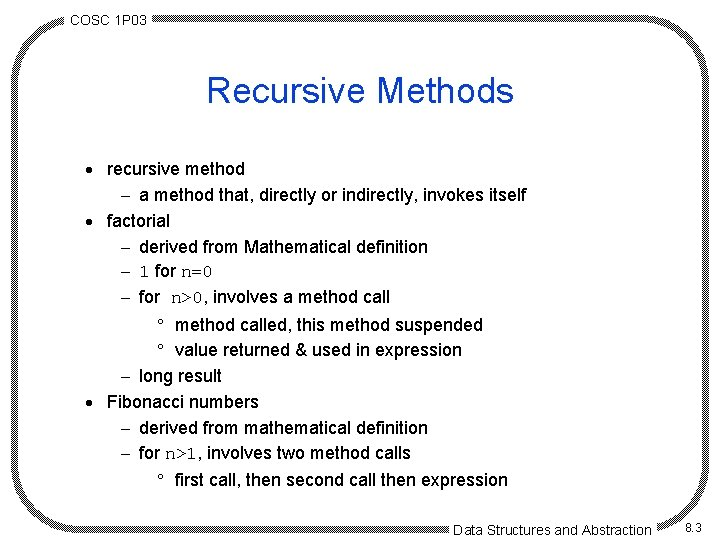COSC 1 P 03 Recursive Methods · recursive method - a method that, directly or indirectly, invokes itself · factorial - derived from Mathematical definition - 1 for n=0 - for n>0, involves a method call ° method called, this method suspended ° value returned & used in expression - long result · Fibonacci numbers - derived from mathematical definition - for n>1, involves two method calls ° first call, then second call then expression Data Structures and Abstraction 8. 3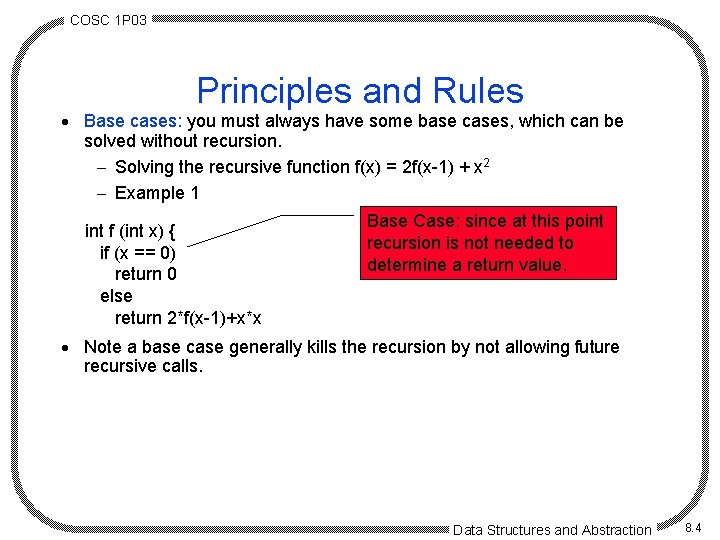COSC 1 P 03 Principles and Rules · Base cases: you must always have some base cases, which can be solved without recursion. - Solving the recursive function f(x) = 2 f(x-1) + x 2 - Example 1 int f (int x) { if (x == 0) return 0 else return 2*f(x-1)+x*x Base Case: since at this point recursion is not needed to determine a return value. · Note a base case generally kills the recursion by not allowing future recursive calls. Data Structures and Abstraction 8. 4COSC 1 P 03 Principles and Rules. Example 2 Head A Print. List (node ptr){ if (ptr != null) { print(ptr. data); Print. List(ptr. next); } } B C D If statement prevents recursive part from being called. Data Structures and Abstraction 8. 5COSC 1 P 03 Principles and Rules. . · Making progress: For the cases that are solved recursively, the recursive call must always be to a case that makes progress toward a base case. int f (int x) { if (x == 0) return 0 else return 2*f(x-1)+x*x We assume that ptr. next will take us toward the end of the list, hence a null pointer. X is reduced toward the base case. Print. List (node ptr){ if (ptr != null) { print(ptr. data); Print. List(ptr. next); } } Data Structures and Abstraction 8. 6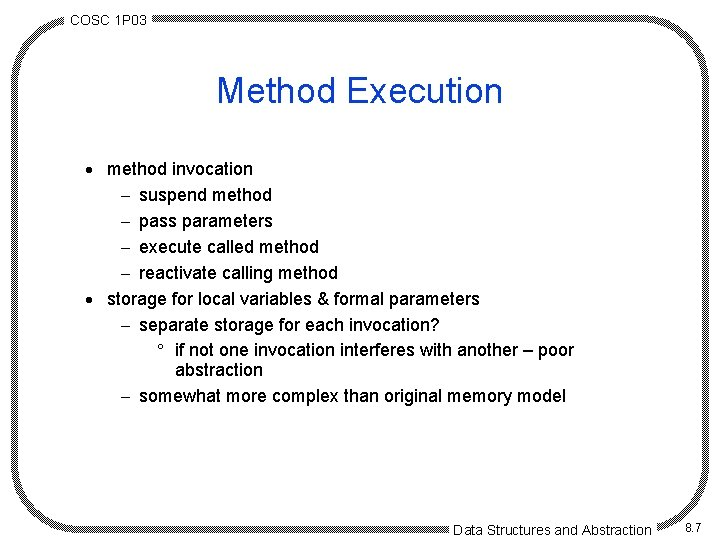COSC 1 P 03 Method Execution · method invocation - suspend method - pass parameters - execute called method - reactivate calling method · storage for local variables & formal parameters - separate storage for each invocation? ° if not one invocation interferes with another – poor abstraction - somewhat more complex than original memory model Data Structures and Abstraction 8. 7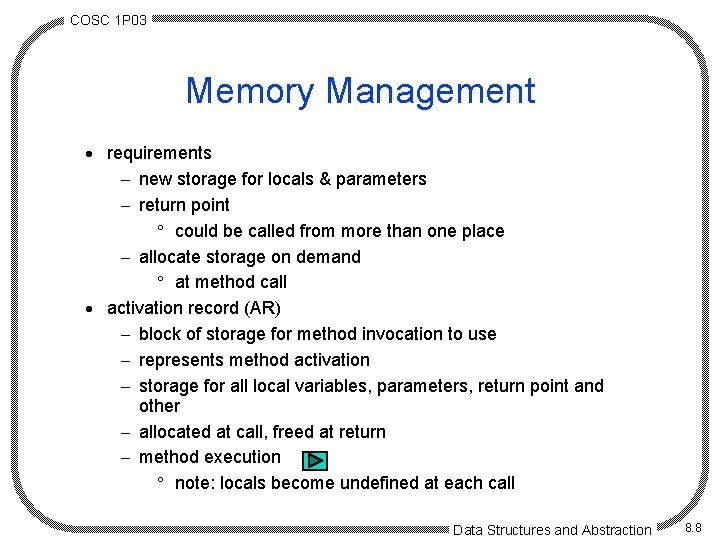COSC 1 P 03 Memory Management · requirements - new storage for locals & parameters - return point ° could be called from more than one place - allocate storage on demand ° at method call · activation record (AR) - block of storage for method invocation to use - represents method activation - storage for all local variables, parameters, return point and other - allocated at call, freed at return - method execution ° note: locals become undefined at each call Data Structures and Abstraction 8. 8COSC 1 P 03 Activation Record Stack · method call/return pattern - LIFO · ARs are stacked - push at call - pop at return - top AR stack visible · memory allocation - stack represented in an array (memory) ° hardware support - code ° fixed size - stack ° grows/shrinks in method/return call pattern - heap ° dynamic allocation of objects ° may grow/shrink according to allocation demands Data Structures and Abstraction 8. 9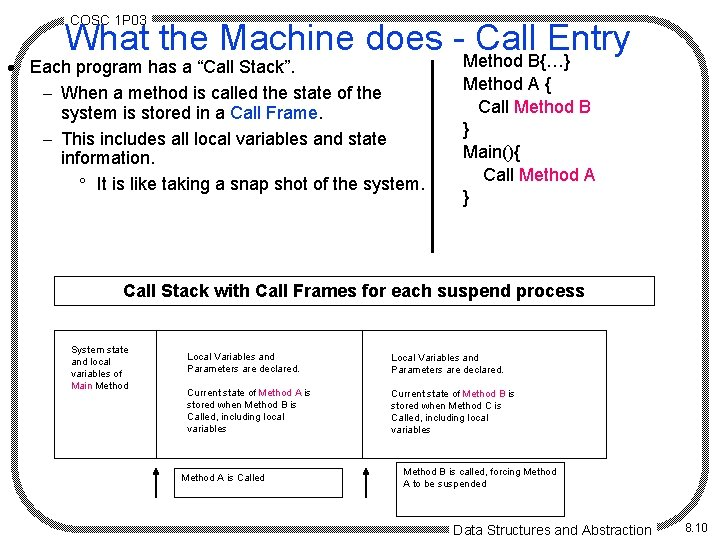COSC 1 P 03 What the Machine does -Method Call. B{…} Entry · Each program has a “Call Stack”. - When a method is called the state of the system is stored in a Call Frame. - This includes all local variables and state information. ° It is like taking a snap shot of the system. Method A { Call Method B } Main(){ Call Method A } Call Stack with Call Frames for each suspend process System state and local variables of Main Method Local Variables and Parameters are declared. Current state of Method A is stored when Method B is Called, including local variables Current state of Method B is stored when Method C is Called, including local variables Method A is Called Method B is called, forcing Method A to be suspended Data Structures and Abstraction 8. 10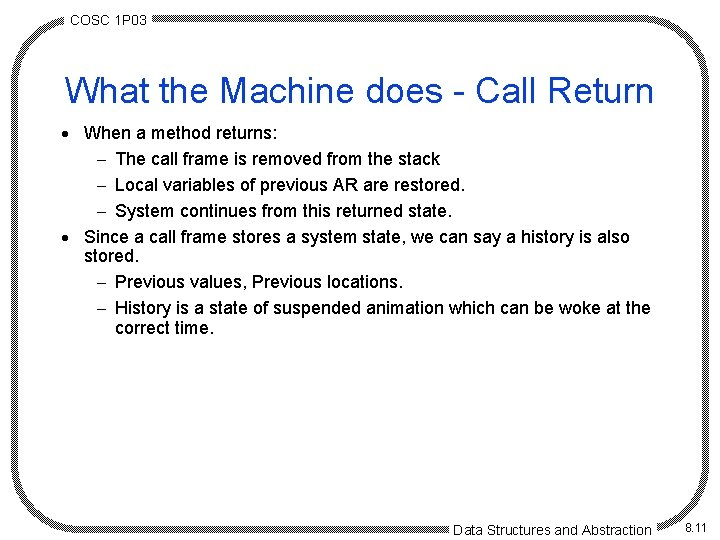COSC 1 P 03 What the Machine does - Call Return · When a method returns: - The call frame is removed from the stack - Local variables of previous AR are restored. - System continues from this returned state. · Since a call frame stores a system state, we can say a history is also stored. - Previous values, Previous locations. - History is a state of suspended animation which can be woke at the correct time. Data Structures and Abstraction 8. 11COSC 1 P 03 Example of Recursion Print. List (node ptr){ if (ptr != null) { print(ptr. data); Print. List(ptr. next); print(ptr. data); } } We wish to print the list forward and backward using recursion. Head A B C D The result of the code would be: ABCDDCBA Data Structures and Abstraction 8. 12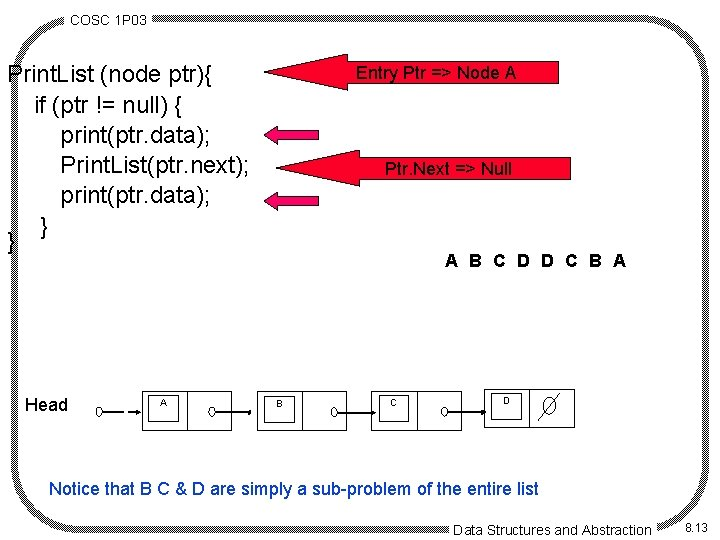COSC 1 P 03 Print. List (node ptr){ if (ptr != null) { print(ptr. data); Print. List(ptr. next); print(ptr. data); } } Head A Entry Ptr => Node A Ptr. Next =>=> Node Null D C B A B C D Notice that B C & D are simply a sub-problem of the entire list Data Structures and Abstraction 8. 13COSC 1 P 03 Pattern of Recursive Execution · ARs for recursive methods handled same as non-recursive - may be more than one AR for the same method on the stack - top AR is for the currently executing invocation of the method · e. g. factorial - initial call (factorial(n)) ° say n=3 - first invocation begins - first recursive call - 2 more recursive calls - just before first return - after first return - 2 more returns - return to main · understanding recursion - abstraction Data Structures and Abstraction 8. 14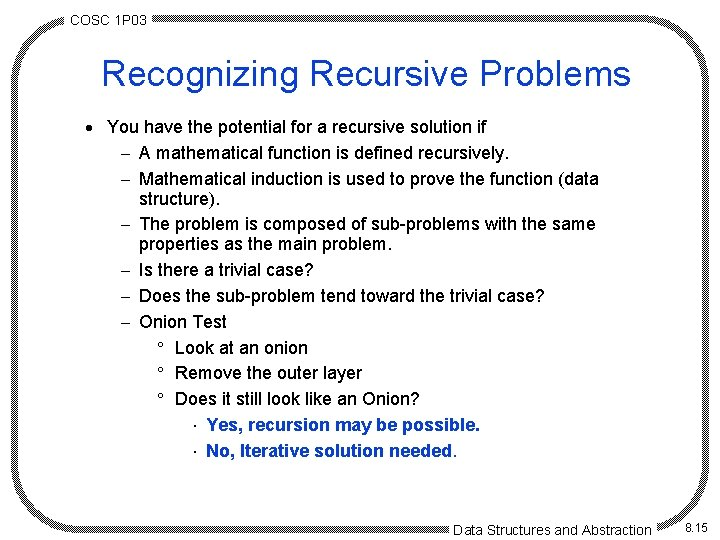COSC 1 P 03 Recognizing Recursive Problems · You have the potential for a recursive solution if - A mathematical function is defined recursively. - Mathematical induction is used to prove the function (data structure). - The problem is composed of sub-problems with the same properties as the main problem. - Is there a trivial case? - Does the sub-problem tend toward the trivial case? - Onion Test ° Look at an onion ° Remove the outer layer ° Does it still look like an Onion? × Yes, recursion may be possible. × No, Iterative solution needed. Data Structures and Abstraction 8. 15COSC 1 P 03 Recursive Algorithms · termination requirements - trivial case - reduction - proof reduction leads to trivial case · proof of termination - factorial ° trivial case: n=0 ° reduction: factorial(n-1) ° proof - Fibonacci numbers ° trivial cases: n=0, n=1 ° reduction: fibonacci(n-1), fibonacci(n-2) ° proof Data Structures and Abstraction 8. 16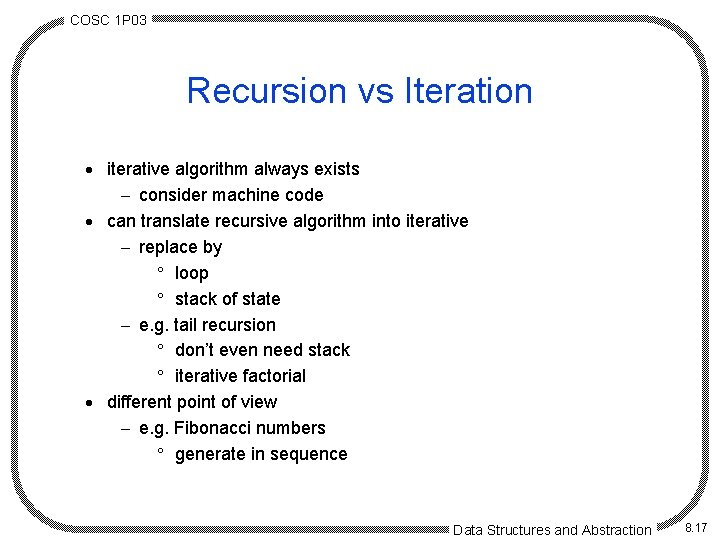COSC 1 P 03 Recursion vs Iteration · iterative algorithm always exists - consider machine code · can translate recursive algorithm into iterative - replace by ° loop ° stack of state - e. g. tail recursion ° don’t even need stack ° iterative factorial · different point of view - e. g. Fibonacci numbers ° generate in sequence Data Structures and Abstraction 8. 17COSC 1 P 03 Examples · Koch curve - space filling curve - transformation of a straight line - order & length - recursion ° 4 Koch curves of lower order - Koch snowflake · image recognition - e. g. MRI - image ° array of cells - locate single connected area - neighbors - avoiding duplication - algorithm and output · Site with lots of good examples Data Structures and Abstraction 8. 18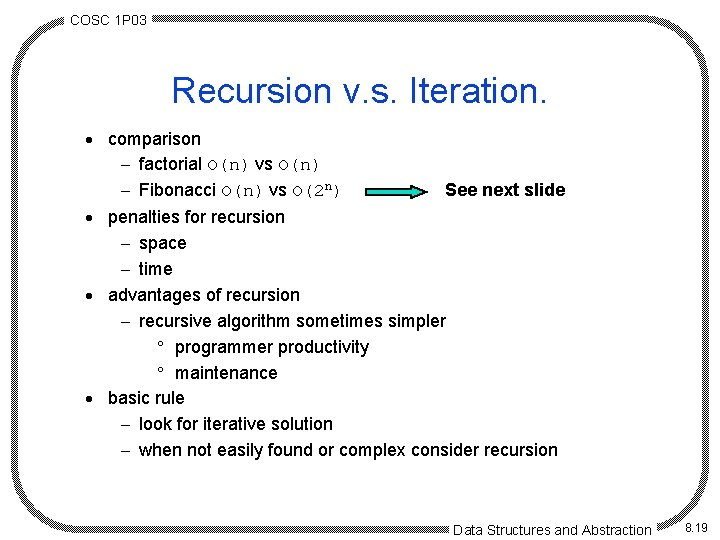COSC 1 P 03 Recursion v. s. Iteration. · comparison - factorial O(n) vs O(n) - Fibonacci O(n) vs O(2 n) See next slide · penalties for recursion - space - time · advantages of recursion - recursive algorithm sometimes simpler ° programmer productivity ° maintenance · basic rule - look for iterative solution - when not easily found or complex consider recursion Data Structures and Abstraction 8. 19COSC 1 P 03 Fibonacci Numbers · n = 3 the first instance of fib calculates: - fib(n-1) ==> [fib(2) and [fib(n-2) ==> fib(1)]] · fib(2) results in instances - fib(1) and fib(0) line 2 · Notice: - Instance of fib(1) is now known form line 2 - But fib(1) in line 1 has yet to be processed - So fib(1) is recalculated. · Results in: - Slow algorithms - Increases the magnitude unnecessarily. line 1 Data Structures and Abstraction 8. 20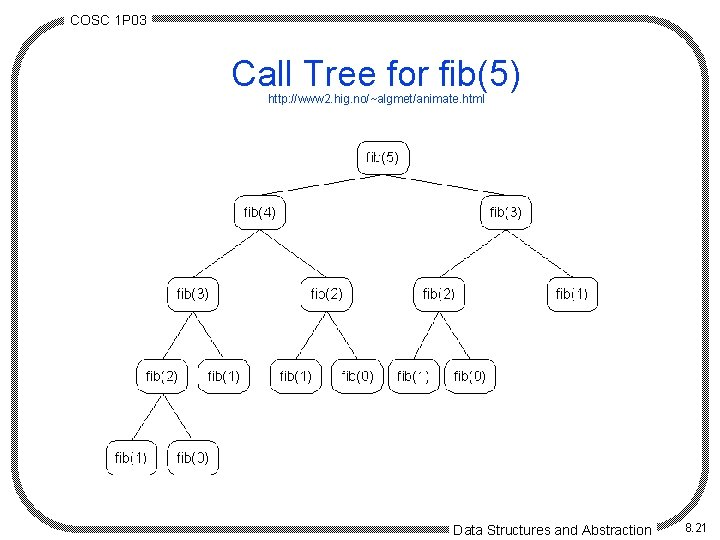COSC 1 P 03 Call Tree for fib(5) http: //www 2. hig. no/~algmet/animate. html Data Structures and Abstraction 8. 21COSC 1 P 03 Design Rule · Design rule: Assume that all recursive calls work. - In general the machine uses its own stack to organize the calls. - Very difficult to trace the calls. - Important to ensure the code adheres to the first two principles, this rule then takes care of itself. Data Structures and Abstraction 8. 22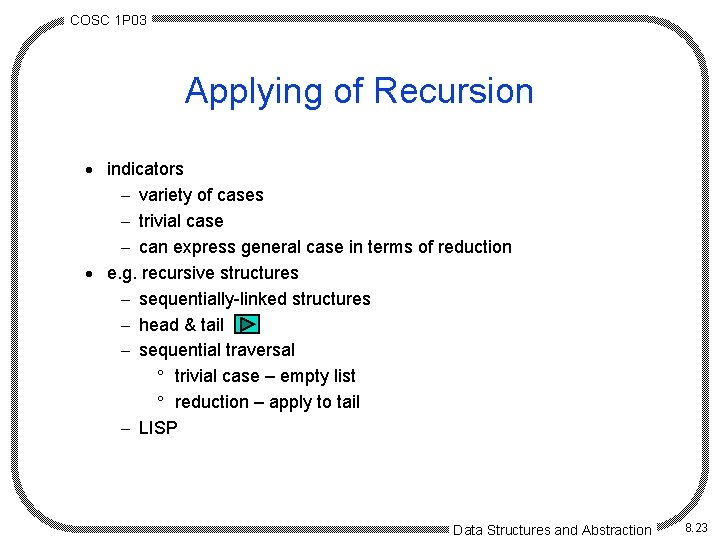COSC 1 P 03 Applying of Recursion · indicators - variety of cases - trivial case - can express general case in terms of reduction · e. g. recursive structures - sequentially-linked structures - head & tail - sequential traversal ° trivial case – empty list ° reduction – apply to tail - LISP Data Structures and Abstraction 8. 23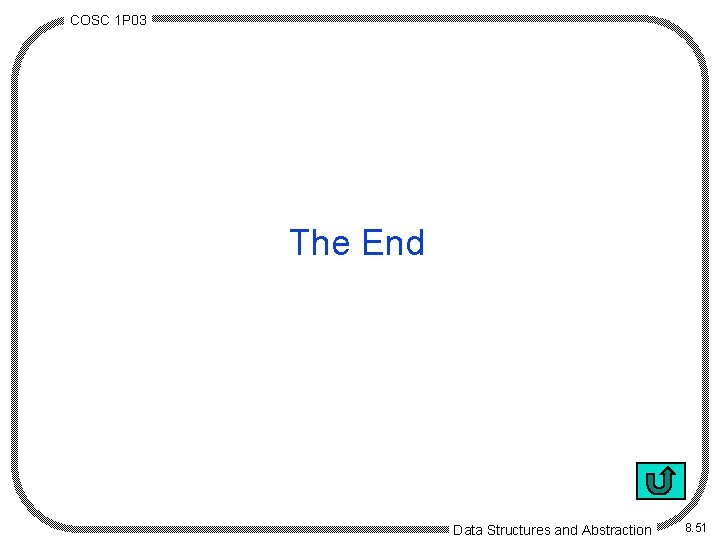COSC 1 P 03 The End Data Structures and Abstraction 8. 51